# Differentiating nuclei according to signal intensity#

A common bio-image analysis task is differentiating cells according to their signal expression. In this example we take a two-channel image of nuclei which express Cy3 and eGFP. Visually, we can easily see that some nuclei expressing Cy3 also express eGFP, others don’t. This notebook demonstrates how to differentiate nuclei segmented in one channel according to the intensity in the other channel.

import pyclesperanto_prototype as cle
import numpy as np
import matplotlib.pyplot as plt
import pandas as pd

cle.get_device()

<Intel(R) Iris(R) Xe Graphics on Platform: Intel(R) OpenCL HD Graphics (1 refs)>


We’re using a dataset published by Heriche et al. licensed CC BY 4.0 available in the Image Data Resource.

# load file
raw_image = imread('../../data/plate1_1_013 [Well 5, Field 1 (Spot 5)].png')

# visualize
imshow(raw_image)

<matplotlib.image.AxesImage at 0x1dac72d7c40>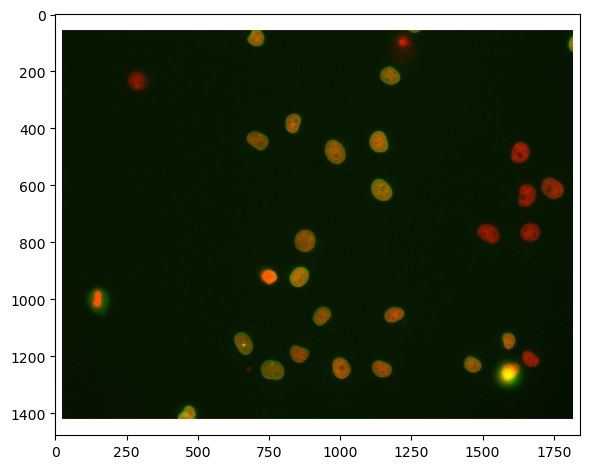First, we need to split channels (read more). After that, we can actually see that not all cells marked with Cy3 (channel 0) are also marked with eGFP (channel 1):

# extract channels
channel_cy3 = raw_image[...,0]
channel_egfp = raw_image[...,1]

# visualize
fig, axs = plt.subplots(1, 2, figsize=(15,15))
axs.imshow(channel_cy3, cmap='gray')
axs.imshow(channel_egfp, cmap='gray')

<matplotlib.image.AxesImage at 0x1dac7328970>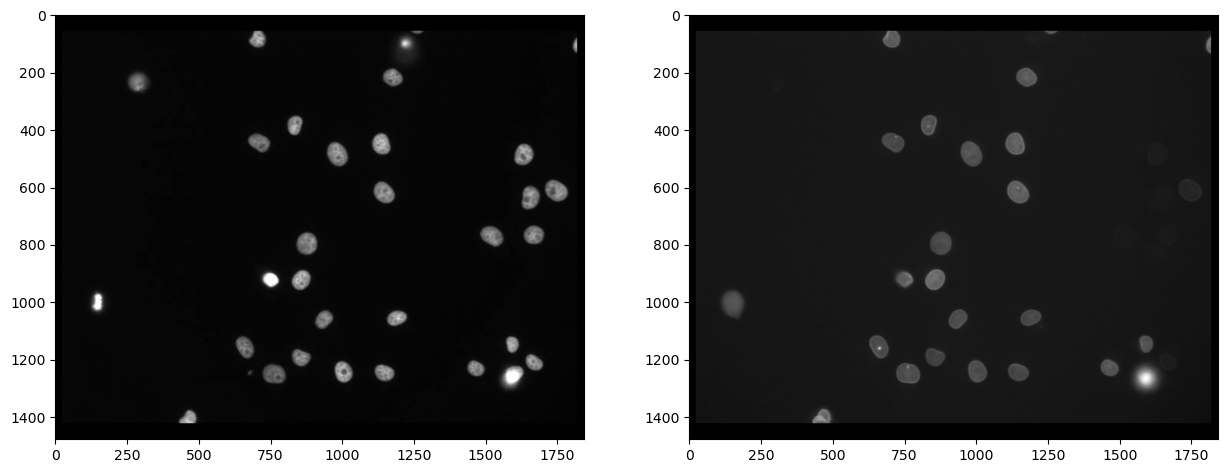## Segmenting nuclei#

As the staining marks the nuclei in the Cy3 channel, it is reasonable to segment nuclei in this channel and afterwards measure the intensity in the other channel. We use Voronoi-Otsu-Labeling for the segmentation because it is a quick and straightforward approach.

# segmentation
nuclei_cy3 = cle.voronoi_otsu_labeling(channel_cy3, spot_sigma=20)

# visualize
fig, axs = plt.subplots(1, 2, figsize=(15,15))
cle.imshow(channel_cy3, plot=axs, color_map="gray")
cle.imshow(nuclei_cy3, plot=axs, labels=True)

C:\Users\rober\miniconda3\envs\bio39\lib\site-packages\pyclesperanto_prototype\_tier9\_imshow.py:46: UserWarning: The imshow parameter color_map is deprecated. Use colormap instead.
warnings.warn("The imshow parameter color_map is deprecated. Use colormap instead.")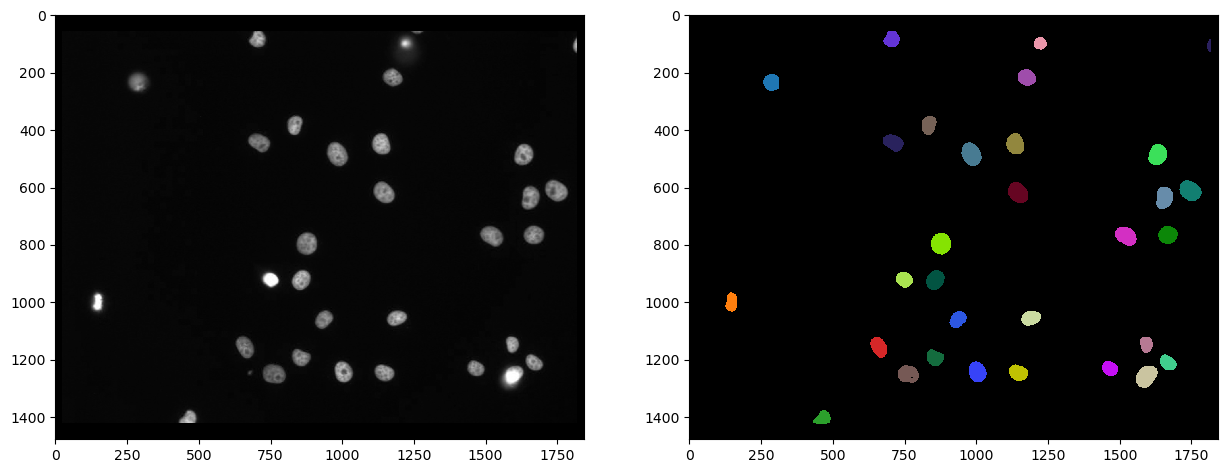Firstly, we can measure the intensity in the second channel, marked with eGFP and visualize that measurement in a parametric image. In such a parametric image, all pixels inside a nucleus have the same value, in this case the mean average intensity of the cell.

intensity_map = cle.mean_intensity_map(channel_egfp, nuclei_cy3)

# visualize
fig, axs = plt.subplots(1, 2, figsize=(15,15))
cle.imshow(channel_egfp, plot=axs, color_map="gray")
cle.imshow(intensity_map, plot=axs, color_map="gray")

C:\Users\rober\miniconda3\envs\bio39\lib\site-packages\pyclesperanto_prototype\_tier9\_imshow.py:46: UserWarning: The imshow parameter color_map is deprecated. Use colormap instead.
warnings.warn("The imshow parameter color_map is deprecated. Use colormap instead.")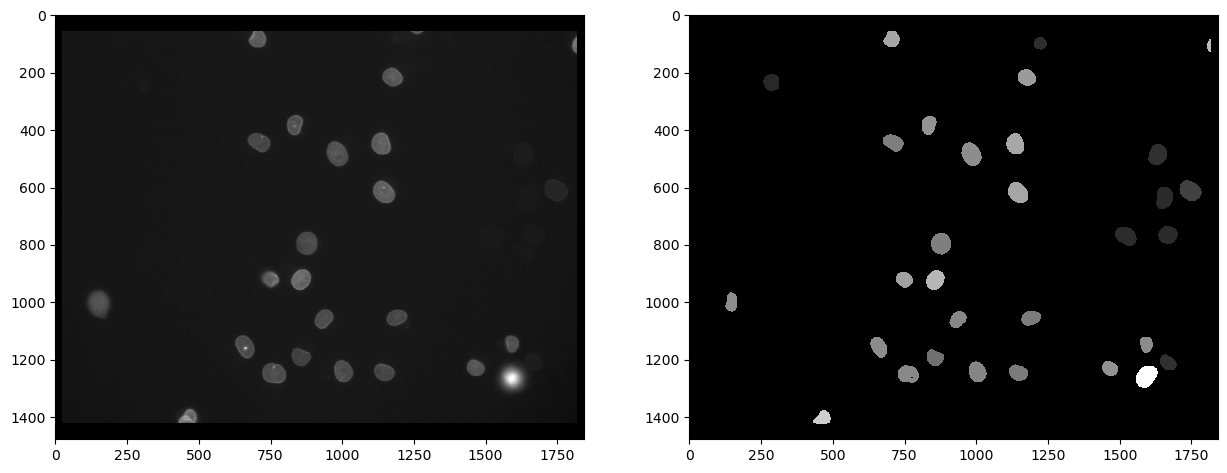From such a parametric image, we can retrieve the intensity values and get them in a vector. The first item in this list has value 0 and corresponds to the intensity of the background, which is 0 in the parametric image.

intensity_vector = cle.read_intensities_from_map(nuclei_cy3, intensity_map)
intensity_vector

cle.array([[  0.        80.875854  23.529799 118.17817   80.730255  95.55177
72.84752   92.34759   78.84362   85.400444 105.108025  65.06639
73.69295   77.40091   81.48371   77.12868   96.58209   95.94536
70.883995  89.70502   72.01046   27.257877  84.460075  25.49711
80.69057  147.49736   28.112642  25.167627  28.448263  25.31705
38.072815 108.81613 ]], dtype=float32)

There is by the way also an alternative way to come to the mean intensities directly, by measuring all properties of the nuclei including position and shape. It the statistics can be further processed as pandas DataFrame.

statistics = cle.statistics_of_background_and_labelled_pixels(channel_egfp, nuclei_cy3)

statistics_df = pd.DataFrame(statistics)

label original_label bbox_min_x bbox_min_y bbox_min_z bbox_max_x bbox_max_y bbox_max_z bbox_width bbox_height ... centroid_z sum_distance_to_centroid mean_distance_to_centroid sum_distance_to_mass_center mean_distance_to_mass_center standard_deviation_intensity max_distance_to_centroid max_distance_to_mass_center mean_max_distance_to_centroid_ratio mean_max_distance_to_mass_center_ratio
0 1 0 0.0 0.0 0.0 1841.0 1477.0 0.0 1842.0 1478.0 ... 0.0 1.683849e+09 640.648682 1.683884e+09 640.661682 8.487288 1187.033203 1187.743164 1.852861 1.853932
1 2 1 127.0 967.0 0.0 167.0 1033.0 0.0 41.0 67.0 ... 0.0 4.128044e+04 18.704323 4.128327e+04 18.705606 4.734930 34.280727 34.338104 1.832770 1.835712
2 3 2 259.0 205.0 0.0 314.0 265.0 0.0 56.0 61.0 ... 0.0 5.392080e+04 19.715099 5.393993e+04 19.722092 1.663603 32.079941 31.469477 1.627176 1.595646
3 4 3 432.0 1377.0 0.0 492.0 1423.0 0.0 61.0 47.0 ... 0.0 3.630314e+04 17.769527 3.636823e+04 17.801388 24.842560 36.856213 36.085457 2.074125 2.027115
4 5 4 631.0 1123.0 0.0 690.0 1194.0 0.0 60.0 72.0 ... 0.0 6.753254e+04 21.686750 6.755171e+04 21.692907 17.358543 38.805695 38.417568 1.789373 1.770974

5 rows × 37 columns

The intensity vector can then be retrieved from the tabular statistics. Note: In this case, the background intensity is not 0, because we were directly reading it from the original image.

intensity_vector2 = statistics['mean_intensity']
intensity_vector2

array([ 20.829758,  80.875854,  23.529799, 118.17817 ,  80.730255,
95.55177 ,  72.84752 ,  92.34759 ,  78.84362 ,  85.400444,
105.108025,  65.06639 ,  73.69295 ,  77.40091 ,  81.48371 ,
77.12868 ,  96.58209 ,  95.94536 ,  70.883995,  89.70502 ,
72.01046 ,  27.257877,  84.460075,  25.49711 ,  80.69057 ,
147.49736 ,  28.112642,  25.167627,  28.448263,  25.31705 ,
38.072815, 108.81613 ], dtype=float32)


To get an averview about the mean intensity measurement, we can use matplotlib to plot a histogram. We ignore the first element, because it corresponds to the background intensity.

plt.hist(intensity_vector2[1:])

(array([ 7.,  1.,  0.,  4., 10.,  5.,  2.,  1.,  0.,  1.]),
array([ 23.52979851,  35.92655563,  48.32331085,  60.72006607,
73.11682129,  85.51358032,  97.91033936, 110.30709076,
122.70384979, 135.1006012 , 147.49736023]),
<BarContainer object of 10 artists>)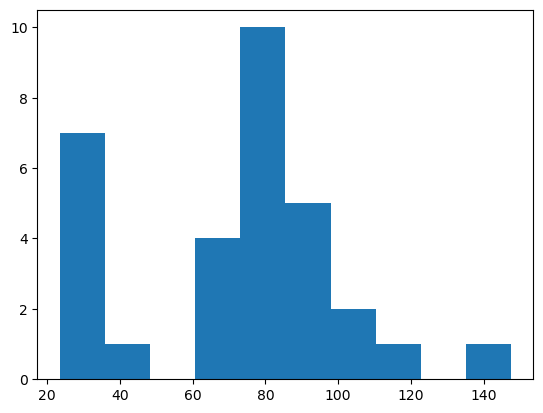From such a histogram, we could conclude that objects with intensity above 50 are positive.

## Selecting labels above a given intensity threshold#

We next generate a new labels image with the nuclei having intensity > 50. Note, all the above steps for extracting the intensity vector are not necessary for this. We just did that to get an idea about a good intensity threshold.

The following label image show the nuclei segmented in the Cy3 channel which have a high intensity in the eGFP channel.

intensity_threshold = 50

nuclei_with_high_intensity_egfg = cle.exclude_labels_with_map_values_within_range(intensity_map, nuclei_cy3, maximum_value_range=intensity_threshold)
intensity_map = cle.mean_intensity_map(channel_egfp, nuclei_cy3)

# visualize
fig, axs = plt.subplots(1, 2, figsize=(15,15))
cle.imshow(channel_egfp, plot=axs, color_map="gray")
cle.imshow(nuclei_with_high_intensity_egfg, plot=axs, labels=True)

C:\Users\rober\miniconda3\envs\bio39\lib\site-packages\pyclesperanto_prototype\_tier9\_imshow.py:46: UserWarning: The imshow parameter color_map is deprecated. Use colormap instead.
warnings.warn("The imshow parameter color_map is deprecated. Use colormap instead.")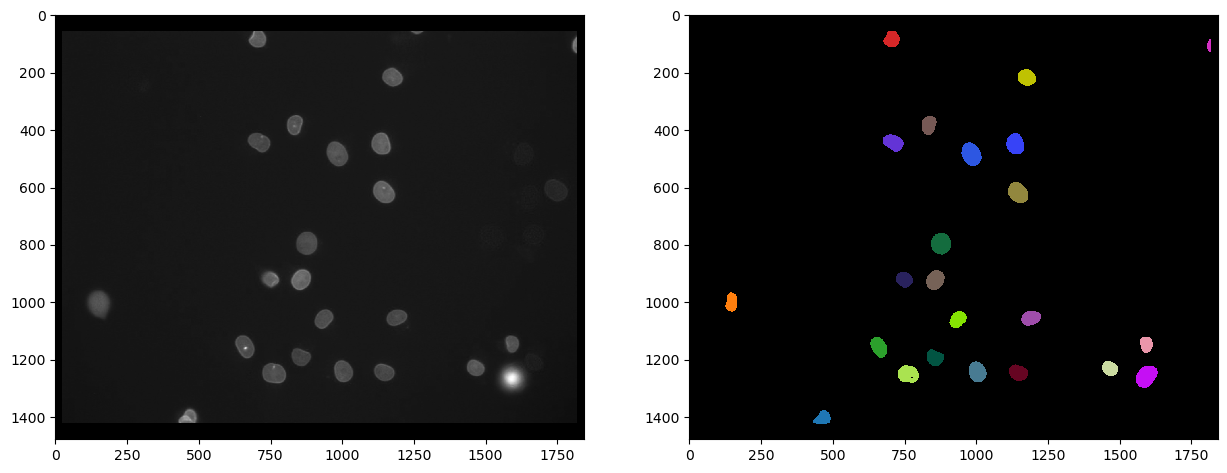And we can also count those by determining the maximum intensity in the label image:

number_of_double_positives = nuclei_with_high_intensity_egfg.max()
print("Number of Cy3 nuclei that also express eGFP", number_of_double_positives)

Number of Cy3 nuclei that also express eGFP 23.0